﻿ 20-sim webhelp > Library > Iconic Diagrams > Mechanical > Translation > 2DSmallRotation > TwoDPoint

# TwoDPoint

Navigation:  Library > Iconic Diagrams > Mechanical > Translation > 2DSmallRotation >

# TwoDPoint## Library

Iconic Diagrams\Mechanical\Translation\2DSmallAngles

X

Y

theta

XY

## Use

Domains: Continuous. Size: 1-D/2-D. Kind: Iconic Diagrams (1D Translation, 2D Planar).

## Description - X

TwoDPoint models are used to connect 1D models to TwoDBodies. They indicate a 1D force or torque that is applied in a certain direction, onto a body. The TwoDPoint-X model forms the connection between the single degree of freedom in x-direction and the center of mass of a body. Consider the example below, where force F in x-direction (single degree of freedom) is pushing upon a body (three degrees of freedom) at an offset YP from the body center of mass.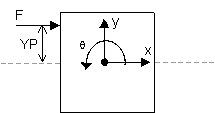To create such a construct the TwoDPoint-X model is used: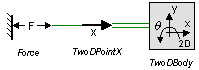The TwoDPoint-X model describes the transformation of force in x-direction to a force in three degrees of freedom. The single degree of freedom port p_in describes the connection in x-direction and the three three degree of freedom port P_out describes connection with the 2D-body (x, y and q).

 P_out.F = p_in.F; P_out.F = 0; P_out.F = -p_in.F * YP;   p_in.v = P_out.v - YP * P_out.v; // x-direction // y-direction // rotation   // x-direction

## Interface - X

 Ports Description p_in P_out 1D Translation port (x). 2D Port with three degrees of freedom (x, y, q). Causality fixed force out P_out fixed velocity out p_in Parameters YP Distance (y-direction) between connection and center of mass [m].

## Description - Y

TwoDPoint models are used to connect 1D models to TwoDBodies. They indicate a 1D force or torque that is applied in a certain direction, onto a body. The TwoDPoint-Y model forms the connection between the single degree of freedom in y-direction and the center of mass of a body. Consider the example below, where force F in y-direction (single degree of freedom) is pushing upon a body (three degrees of freedom) at an offset XP from the body center of mass.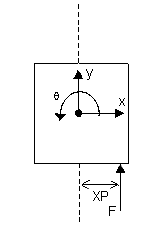To create such a construct the 2D-point-Y model is used: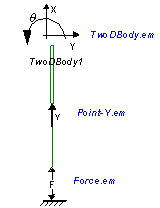The 2D-point-Y model describes the transformation of force in y-direction to a force in three degrees of freedom. The single degree of freedom port p_in describes the connection in y-direction and the three three degree of freedom port P_out describes connection with the 2D-body (x, y and q).

 P_out.F = 0; P_out.F = p_in.F; P_out.F = p_in.F * XP;   p_in.v = P_out.v + XP * P_out.v; // x-direction // y-direction // rotation   // y-direction

## Interface - Y

 Ports Description p_in P_out 1D Translation port (y). 2D Port with three degrees of freedom (x, y, q). Causality fixed force out P_out fixed velocity out p_in Parameters XP Distance (x-direction) between connection and center of mass [m].

## Description - theta

TwoDPoint models are used to connect 1D models to TwoDBodies. They indicate a 1D force or torque that is applied in a certain direction, onto a body. The TwoDPoint-Theta model forms the connection between the single degree of freedom rotation and the center of mass of a body. Consider the example below, where torque (single degree of freedom) is acting on a body (three degrees of freedom).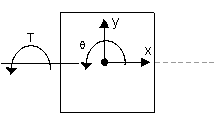To create such a construct the TwoDPoint-Theta model is used: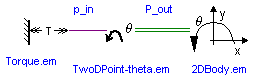The TwoDPoint-Theta model describes the transformation of a single rotation to a rotation of the 2D-body. The single degree of freedom port p_in describes the connection to the single degree rotation the three three degree of freedom port P_out describes connection with the 2D-body (x, y and q).

 P_out.F = 0; P_out.F = 0; P_out.F = p_in.T;   p_in.omega = P_out.v; // x-direction // y-direction // rotation   // rotation

## Interface - theta

 Ports Description p_in P_out 1D Rotation port (q). 2D Port with three degrees of freedom (x, y, q). Causality fixed force out P_out fixed velocity out p_in

## Description - XY

TwoDPoint models are used to connect 1D models to TwoDBodies. They indicate a 1D force or torque that is applied in a certain direction, onto a body. The TwoDPoint-XY model forms the connection between a single degree of freedom in arbitrary direction and the center of mass of a body. Consider the example below, where force F (single degree of freedom) is pushing upon a body (three degrees of freedom) with an arbitrary angle theta at an offset XP and YP from the body center of mass.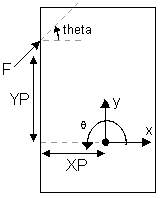To create such a construct the TwoDPoint-XY model is used: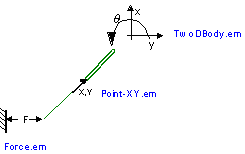The TwoDPoint-XY model describes the transformation of a one degree of freedom force in an arbitrary direction to a force in three degrees of freedom. The single degree of freedom port p_in describes the connection in the arbitrary direction and the three three degree of freedom port P_out describes connection with the 2D-body (x, y and q).

 Fx = cos(theta)*p_in.F; Fy = sin(theta)*p_in.F;   P_out.F = Fx; P_out.F = Fy; P_out.F = Fy * XP - Fx * YP;   p_in.v = cos(theta)*P_out.v + sin(theta)*P_out.v + XP * sin(theta)* P_out.v - YP * cos(theta) * P_out.v; // arbitrary-direction // arbitrary-direction   // x-direction // y-direction // rotation   // arbitrary-direction

## Interface - XY

 Ports Description p_in P_out 1D Translation port in arbitrary direction. 2D Port with three degrees of freedom (x, y, q). Causality fixed force out P_out fixed velocity out p_in Parameters YP XP theta Distance (y-direction) between connection and center of mass [m]. Distance (x-direction) between connection and center of mass [m]. Angle of impact of the single degree of freedom port [rad].

## Note

 • A TwoDBody has to be connected to at least three unequal TwoDPoint models to prevent it from free motion (rotation & translation).
 • Flipping or rotating the model does not change the direction of applied forces or measured directions. Preferably leave the orientation as it pops up on the screen.